# RD Sharma Solutions Chapter 2 Polynomials Exercise 2.3 Class 10 Maths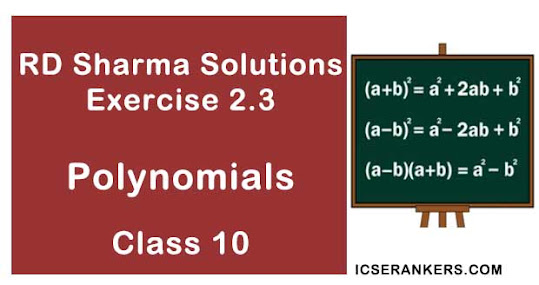Chapter Name RD Sharma Chapter 2 Polynomials Book Name RD Sharma Mathematics for Class 10 Other Exercises Exercise 2.1Exercise 2.2 Related Study NCERT Solutions for Class 10 Maths

### Exercise 2.3 Solutions

1. Apply division algorithm to find the quotient q(x) and remainder r(x) on dividing f(x) by g(x) in each of the following:
(i) f(x) = x3 - 6x2 + 11x - 6,  g(x) = x2 + x + 1
(ii)  f(x) = 10x4 + 17x3 - 62x2  + 30x - 105,  g(x) = 2x2 + 7x + 1
(iii) f(x) = 4x3 + 8x2 + 8x + 7, g(x) = 2x2 - x + 1
(iv) f(x) = 15x3 - 20x2 + 13x - 12;  g(x) = x2 - 2x + 2
Solution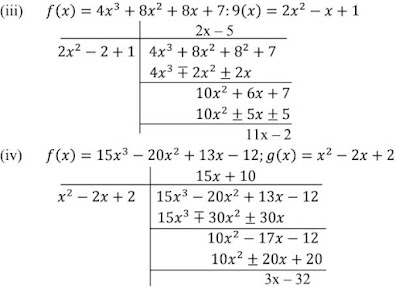3. Obtain all zeros of the polynomial f(x) = 2x4 + x3 - 14x2 - 19x - 6, if two of its zeros are -2 and -1.
Solution

f(x) = 2x4 + x3 - 14x2 - 19x - 6
If the two zeroes of the polynomial are -2 and -1, then its factors are (x+2) and (x+1).
(x+2)(x+1) = x2 + x + 2x = x2 + 3x + 2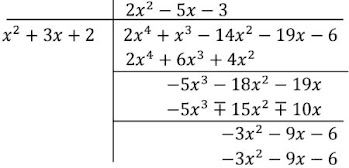∴ 2x4 + x3 - 14x2 - 19x - 6
= (2x2 - 5x - 3)[x2 + 3x + 2]
= [2x + 1][x - 3][x+2][x+1]
∴ zero all x = -1/2, 3, -2, -1

4. Obtain all zeros of f(x) = x3 + 13x2 + 32x + 20, if one of its zeros is -2.
Solution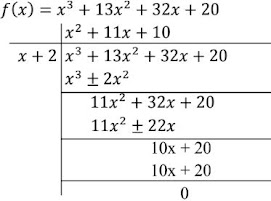(x2 + 11x + 10) = x2 + 10x + x + 20(x + 10) = (x+ 1)(x+10)
∴ The zeroes of the polynomial are -1, -10, -2.

5. Obtain all zeros of the polynomial f(x) = x4 - 3x2 = x2 + 9x - 6 if two of its zeros are -√3, and √3.
Solution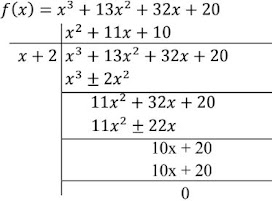(x2 - 3)(x2 - 3x + 2) = (x + √3)(x - √3)(x2 - 2x - x + 2)
= (x + √3)(x - √3)(x-2)(x-2)
Zeroes are - √3, √3, 1, 2

6. Find all zeros of the polynomial f(x) = 2x4 - 2x3 - 7x2 + 3x + 6, if its two zeroes are -√(3/2) and √(3/2).
Solution

If the zeroes of the polynomial are -√(3/2) and √(3/2)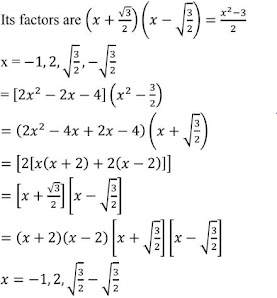7. What must be added to the polynomial f(x) = x4 + 2x3 - 2x2 + x - 1 so that the resulting polynomial is exactly divisible by x2 + 2x -3 ?
Solution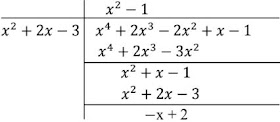we must add x - 2 in order to get the resulting polynomial exactly divisible by x2 + 2x - 3

8. What must be subtracted from the polynomial x4 + 2x3 - 13x2 - 12x + 21, so that the resulting polynomial is exactly divisible by x2 - 4x + 3 ?
Solution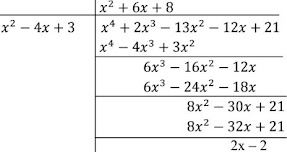We must subtract [2x - 2] + 10m the given polynomial so as to get the resulting polynomial exactly divisible by x2 - x + 3.

9. Find all the zeroes of the polynomial x4 + x3 - 34x2 - 4x + 120, if two of its zeroes are 2 and -2.
Solution
f(x) = x4 + x3 - 34x2 - 4x + 120

⇒ x = -2 is a solution
x = -2 is a factor
x = -2 is a solution

x = +2 is a factor
here,
(x - 2)(x + 2) is a factor of f(x)
x2 - 4 is a factor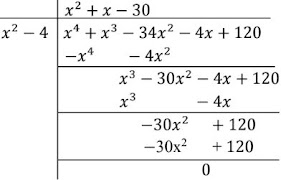Hence, x4 + x3 - 34x2 - 4x + 120 = (x2 -4)(x2 + x -30)
x4 + x3 - 34x2 - 4x + 120 = (x2 - 4)(x2 + 6x - 5x - 30)
⇒ x4 + x3 - 34x2 - 4x + 120 = (x2 -4)[x(x+6) - 5(x + 6)]
⇒ x4 + x3 - 34x2 - 4x + 120 = (x2 -4)(x + 6) (x- 5)
Other zeroes are
x + 6 = 0
⇒ x = -6
x - 5 = 0
⇒ x = 5
Se of zeroes for f(x) [2, -2, 6, 5]

10. Find all zeros of the polynomial 2x4 + 7x3 - 19x2 - 14x + 30,  if two of its zeros are √2 and - √2.
Solution
f(x) = 2x4 + 7x3 - 19x2 - 14x + 30
x = √2 is a solution
x - √2 is a solution
x + √2 is a factor
Here , (x + √2)(x -√2) is a factor of f(x)
x2 - 2 is a factor of f(x)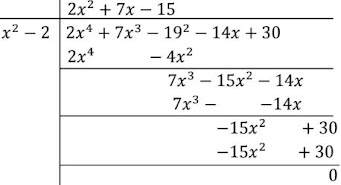Hence, 2x4 + 7x3 - 19x2 - 14x + 30 = (x2 - 2)(2x2 + 7x - 15)
= (x2 - 2)(2x2 + 10x - 3x - 15)
= (x2 - 2)[2x(x+ 5) - 3(x + 5)]
= (x2 - 2)(x + 5)(x - 3)
Other zeroes are :
x + 5 = 0
⇒ x = -5
2x - 3 = 0
⇒ 2x = 3
⇒ x = 3/2
Hence, the set of zeroes for f(x) {-5, 3/2, √2, -√2}

11. Find all the zeroes of the polynomial 2x3 + x2 - 6x - 3,  if two of its zeros are -√3 and √3.
Solution

f(x) =   2x3 + x2 - 6x - 3
x = -√3 is a solution

x + √3 is a factor
x = √3 is a solution
x - √3  is a factor
Here, (x +√3)(x-√3) is a factor of f(x)
x2 - 3 is a factor of f(x)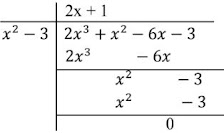Hence, 2x3 + x2 - 6x - 3 = (x2 - 3)(2x - 1)
Other zeroes of f(x) is 2x + 1  = 0
x = -1/2
Set of zeroes {√3, -√3, -1/2}

12. Find all the zeros of the polynomial x3 + 3x2 -2x - 6, if two of its zeros are -√2 and √2.
Solution

Since, -√2 and √2 are zeroes of polynomial f(x) = x3 + 3x2 -2x - 6
(x + √2)(x - √2) = x2 - 2 is a factor of f(x)
Now we divide f(x) = x3 + 3x2 - 2x - 6 by
g(x) = x2 - 2 to b find the other zeroes of f(x)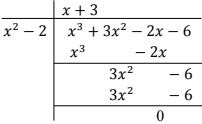By division algorithm, we have
⇒ x3 + 3x2 - 2x - 6 = (x2 - 2)(x+ 3)
⇒ x3 + 3x2 - 2x - 6 = (x + √2)(x - √2(x + 3)
Here the zeroes of the given polynomials are -√2, √2 and -3